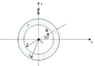# Gauss Law sphere help

mattmannmf
An infinite line of charge with linear density λ = 7.6 μC/m is positioned along the axis of a thick insulating shell of inner radius a = 3 cm and outer radius b = 5 cm. The insulating shell is uniformly charged with a volume density of ρ = -611 μC/m3. (see attachment)

What is Ex(R), the value of the x-component of the electric field at point R, located a distance 1.5 cm along a line that makes an angle of 30o with the x-axis?

What is Ey(R), the value of the y-component of the electric field at point R, located a distance 1.5 cm along a line that makes an angle of 30o with the x-axis?

So I need to find E.

Initially I used this equation:
E= 1/ (4*pi* eo) * (λ/ r) *[either cos/sin counting on which one you're solving for]

where λ = 7.6*10^-6 and r= .015m

The units seem to make sense (comes out to be N/C) but when I get an answer (3945479 for E x-comp) its wrong. Any help please? I'm not really that educated about gauss's law so I am not sure what else to do.

#### Attachments

•h4_cylinder.png
5.1 KB · Views: 502

Homework Helper
Gold Member
Hello mattmannmf,
So I need to find E.
Gauss' law states that

$$\oint _S \vec E \cdot \vec{dA}= \frac{Q_{ENC}}{\epsilon _0}$$

What this means is that the dot product of the electric field and the differential area vector, summed over all area in a closed surface (any arbitrary close surface), is proportional to the total charge enclosed within that surface. More on how to use this idea below.
Initially I used this equation:
E= 1/ (4*pi* eo) * (λ/ r) *[either cos/sin counting on which one you're solving for]
Not quite (but close).In one way, you're on the right track for ignoring the insulating shell, when working with the electric fields inside the shell. So that much is okay. But something wasn't right with your derivation of the above equation (the circumference of a circle is not 4πr). Again, more on the below.
where λ = 7.6*10^-6 and r= .015m

The units seem to make sense (comes out to be N/C) but when I get an answer (3945479 for E x-comp) its wrong. Any help please?

I'm not really that educated about gauss's law so I am not sure what else to do.
Gauss's law is pretty neat, and it can be used all the time for situations. But in the case where you are using it to find the electric field, it's really only useful in a handful of specific situations, and only where symmetry is involved. As a matter of fact, it's really only useful in finding the electric field if you dealing spherical points, shells or spheres (things with spherically symmetrical charge distribution); infinitely long (or at least very long) line charges or cylinder charges (things with cylindrical symmetry), or infinitely long (or at least very long) planes (things with planar charge distribution).

The key to using to find the electric field is to follow these guidelines:
(1) Pick a Gaussian surface such that the electric field is perpendicular to the surface (i.e. parallel to the normal vector of the surface) and the magnitude of the electric field is constant all across the entire surface. (You can ignore the caps of cylinders [and/or sides of 'pillboxes'] because the contribution is negligible when considering infinitely cylinders and planes, and secondly because the electric flux through the caps of a Gaussian cylinder is zero [dot product of the vectors is zero] for the caps anyway, when considering infinitely long line and cylinder charges.)
(2) If you've followed the first step, the integral is a snap, because $\oint E \cdot dA = EA$ if the conditions in guideline 1) are met.
(3) Solve for the magnitude of E.